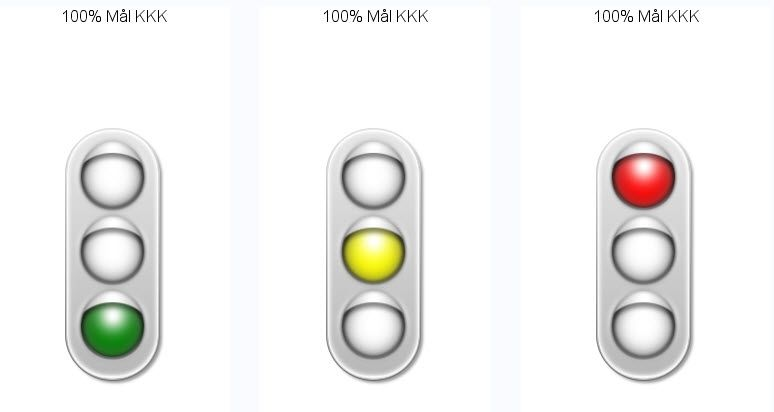## GKPI side by side on the web/Stored Prcoes

Hi,

I am trying to put three KPIs created by Proc GPKI - but I want them placed side-by-side. I can get the desired result when I

out it to a ods html destination, but how does I force it to be display on the web when the users run the Stored Proces? Thanks in advance.

********************************************************************;

Here is some  of my code:

%let panelcolumns = 3;
%let panelborder = 1;
%let embedded_titles = no;

goptions reset=all device=javaimg xpixels=440 ypixels=150 ;

filename odsout "c:\temp" ;

title1 'Using PROC GKPI with the HTMLPANEL Tagset';

ods listing close;

ods tagsets.htmlpanel
path = odsout
body = "paneltest.html" (title="HTMLPANEL Test")
nogtitle
nogfootnote
style=minimal;

ods tagsets.htmlpanel event=panel(start);

proc gkpi mode=raised;
trafficlight actual=&maal100o. bounds=(100 97 85 0) /colors=(green yellow red)  noavalue
lfont=(h=16pt) bfont=(h=16pt) afont=(h=20pt)   label="100% Mål KKK" ;
run;

proc gkpi mode=raised;
trafficlight actual=&maal100p. bounds=(100 97 85 0) /colors=(green yellow red)  noavalue
lfont=(h=16pt) bfont=(h=16pt) afont=(h=20pt)  label="100% Mål YYY";
run;
quit;

proc gkpi mode=raised;
trafficlight actual=&maal100p. bounds=(100 97 85 0) /colors=(green yellow red)  noavalue
lfont=(h=16pt) bfont=(h=16pt) afont=(h=20pt)  label="100% XXXX";
run;
quit;

ods tagsets.htmlpanel event=panel(finish);
ods _all_ close;
ods listing;

1 ACCEPTED SOLUTION

Accepted Solutions

## Re: GKPI side by side on the web/Stored Prcoes

You can use the ODS LAYOUT feature for HTML based output

See this code sample, it will create three KPI's side by side

``````ods layout gridded COLUMNS=3
width=50pct
;
ods region;
proc gkpi mode=raised;
trafficlight actual=100 bounds=(100 97 85 0) /
colors=(green yellow red)  noavalue
lfont=(h=16pt) bfont=(h=16pt) afont=(h=20pt)   label="100% Mål KKK"
;
run;
ods region;
proc gkpi mode=raised;
trafficlight actual=96 bounds=(100 97 85 0) /
colors=(green yellow red)  noavalue
lfont=(h=16pt) bfont=(h=16pt) afont=(h=20pt)   label="100% Mål KKK"
;
run;
ods region;
proc gkpi mode=raised;
trafficlight actual=75 bounds=(100 97 85 0) /
colors=(green yellow red)  noavalue
lfont=(h=16pt) bfont=(h=16pt) afont=(h=20pt)   label="100% Mål KKK"
;
run;
ods layout end;``````

Bruno

3 REPLIES 3

## Re: GKPI side by side on the web/Stored Prcoes

You can use the ODS LAYOUT feature for HTML based output

See this code sample, it will create three KPI's side by side

``````ods layout gridded COLUMNS=3
width=50pct
;
ods region;
proc gkpi mode=raised;
trafficlight actual=100 bounds=(100 97 85 0) /
colors=(green yellow red)  noavalue
lfont=(h=16pt) bfont=(h=16pt) afont=(h=20pt)   label="100% Mål KKK"
;
run;
ods region;
proc gkpi mode=raised;
trafficlight actual=96 bounds=(100 97 85 0) /
colors=(green yellow red)  noavalue
lfont=(h=16pt) bfont=(h=16pt) afont=(h=20pt)   label="100% Mål KKK"
;
run;
ods region;
proc gkpi mode=raised;
trafficlight actual=75 bounds=(100 97 85 0) /
colors=(green yellow red)  noavalue
lfont=(h=16pt) bfont=(h=16pt) afont=(h=20pt)   label="100% Mål KKK"
;
run;
ods layout end;``````

Bruno

## Re: GKPI side by side on the web/Stored Prcoes

Awsiome!!! thanks a lot for your help.  ODS LAYOUT feature is a great new feature in SAS...It looks perfect.

## Re: GKPI side by side on the web/Stored Prcoes

For other readers, a picture is worth a thousand words.  Here's the output of the code from @BrunoMueller:Discussion stats
• 3 replies
• 1088 views
• 2 likes
• 3 in conversation